# Lab 8: Shift Register and Binary

## 1. First Build

It would be a really good idea to build this circuit before going to the lab because it is time consuming to connect all of the wires.

### 1.1 The Circuit

The circuit here is CIRC-05, from your book. For formality, the schematic diagram is the following: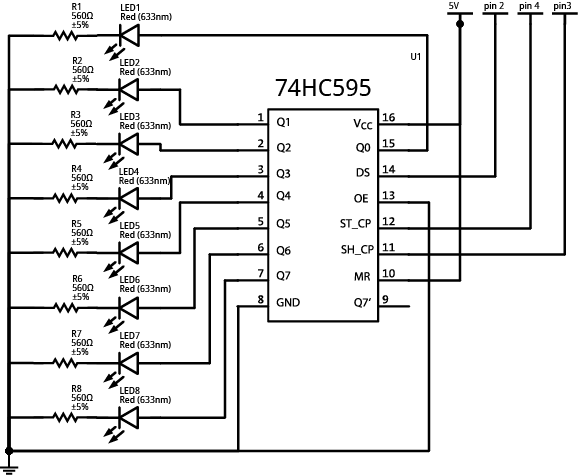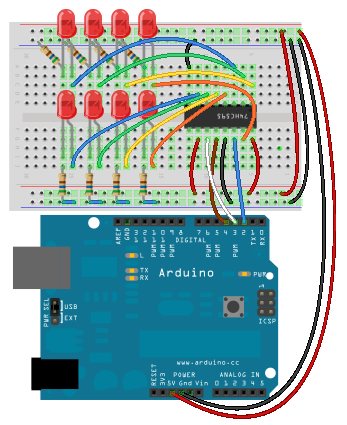Note:

• The shift register has a little notch missing at the "top". That notch on the right in this diagram.

### 1.2 The Code

The code for this circuit is available here: CIRC05-code.txt

You can use `Command-a` to select everything, `Command-c` to copy from your Safari window, and `Command-v` to paste it into your Arduino window.

The code that immediately runs looks like this (notice that some functions are missing):

``````
/*     ---------------------------------------------------------
*     |  Arduino Experimentation Kit Example Code             |
*     |  CIRC-05 .: 8 More LEDs :. (74HC595 Shift Register)   |
*     ---------------------------------------------------------
*
* We have already controlled 8 LEDs however this does it in a slightly
* different manner. Rather than using 8 pins we will use just three
*
*
*/

//Pin Definitions
//Pin Definitions
//The 74HC595 uses a serial communication
int data = 2;
int clock = 3;
int latch = 4;

/*
* setup() - this function runs once when you turn your Arduino on
* We set the three control pins to outputs
*/
void setup()
{
pinMode(data, OUTPUT);
pinMode(clock, OUTPUT);
pinMode(latch, OUTPUT);
}

/*
* loop() - this function will start after setup finishes and then repeat
* we set which LEDs we want on then call a routine which sends the states to the
* 74HC595
*/
void loop()                     // run over and over again
{
int delayTime = 100; //the number of milliseconds to delay between LED updates
for(int i = 0; i < 256; i++){
updateLEDs(i);
delay(delayTime);
}
}

/*
* updateLEDs() - sends the LED states set in ledStates to the 74HC595
* sequence
*/
void updateLEDs(int value){
digitalWrite(latch, LOW);     //Pulls the chips latch low
shiftOut(data, clock, MSBFIRST, value); //Shifts out the 8 bits to the shift register
digitalWrite(latch, HIGH);   //Pulls the latch high displaying the data
}
```
```

## 2. Hardware Theory

If you recall, last lab involved eight LED's and used eight output pins (one for each LED). To expand this circuit to 16 LEDs, you would use 16 output pins. Do you detect a problem with this? (remember we only have 14 output pins).

This week, we have the same number of LED's (eight), but we only use three of the output pins. The magic that makes this happen is the Shift Register. In fact, you can chain shift registers together to control multiples of eight LEDs (for instance 16, 24, 32, etc) and still only use three output pins.

WOW...Let's find out how this magic Shift Register works.

### 2.1 Overview--"Rollover Rollover"

The concept of a shift register, brings to mind the nursery song: http://www.youtube.com/watch?v=WqF0ev8UOB4. Well, it's not quite this idea. But now that you have this ridiculous image in your head, you might remember the concept behind shift registers.

The idea is that a "rollover" occurs and one on the edge falls out. The part that is missing in this idea is that there are others that are added to the other side. Bits (sequences of 1's and 0's) are the things that perform the rolling over. For instance, if we start with a pattern like this:

0 0 0 0 0 1 0 1

and want to end up with this pattern:

0 0 1 1 0 0 1 1

The sequence of "shifts" (the more technical term for "rollovers") could be represented as follows. Notice that the movement of the bits is toward the left.

bit shifted out pattern of bits bit shifted in

0 0 0 0 0 1 0 1

0
0 0 0 0 1 0 1 0
0
0
0 0 0 1 0 1 0 0
0
0
0 0 1 0 1 0 0 1
1
0
0 1 0 1 0 0 1 1
1
0
1 0 1 0 0 1 1 0
0
1
0 1 0 0 1 1 0 0
0
0
1 0 0 1 1 0 0 1
1
1
0 0 1 1 0 0 1 1
1

We can also imagine that there is some "bell" or alarm that triggers this shift to occur. This "bell" is actually referred to as a clock. The change in the clock from LOW to HIGH is the trigger that causes the shift to occur.

### 2.2 What is a Shift Register

A shift register may look complicated, but just remember that ten of the pins are for the pattern (i.e. eight pins for the pattern, one for the shift in, and one for the shift out), two of the pins are for power and ground, and the remaining pins are to control the pattern.

Let's look at a diagram and examine the pieces of a shift register: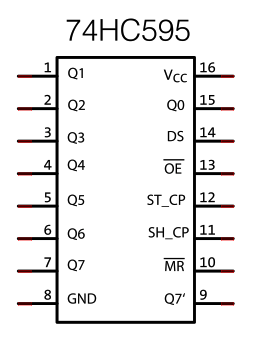The pieces of this diagram that are data related are:

• Q0 to Q7 (pin 1 to 7 and 15) are the eight pins containing the pattern. These pins are also know as the "output pins"
• Q7' (pin 9) is the bit that gets rolled (or shifted) out.
• DS (pin 14) is the bit that is getting rolled (shifted) in.

The pieces that are power and ground related are:

• GND (pin 8) is (you guessed it) connected to ground
• VCC (pin 16) is connected to 5V

The remaining four pins are used to control the pattern in the shift register:

• MR (pin 10) is the "Master Reclear".
• It will "empty" the whole shift register if pulled LOW. Notice that in our starting circuit, this pin is connected to 5V. This is because it must be pulled to HIGH to enable the shift register.
• SH_CP (pin 11) is the "SHift register Clock Pin".
• When this pin is pulled HIGH, it will shift the register. This pin will alternate between HIGH and LOW. In our analogy, this is like the "bell" that indicates that a change will occur.
• ST_CP (pin 12) is the "STorage register Clock Pin".
• Needs to be pulled to HIGH to have the "pattern" of bits be output by the shift register. This will be pulled HIGH after SH_CP has gone LOW. This pin will alternate between HIGH and LOW as well. This pin could be analogous to a "display" trigger. You could have multiple shifts, but only display the ending pattern.
• OE (pin 13) is the "Output Enable" pin.
• This pin enables the output when tied to GND and disables output when HIGH. Notice how our circuit has pin 13 tied to ground.

### 2.3 How the Arduino Controls the Shift Register

In our implementation, we have three pins connecting the Arduino to the shift register:

1. DS (the bit to be shifted in)
2. ST_CP (the clock that controls when the output gets displayed)
3. SH_CP (the clock that controls when the shifting occurs)

These three are related as follows:

When the signal on the SH_CP-pin goes HIGH, all the values get shifted and a new value (from DS) gets shifted in. When you want the "pattern" that has been created to be output, you must also change the ST_CP pin to HIGH. This updates the output-pins with the new data.

If you are interested and would like to see the step by step interaction of these pieces (with the patterns from Section 3.1), some code is available for you in this file: shift_ Clocks.html

## 3. Software Theory

### 3.1 The `shiftOut()` Function

Let us take a look at the partial code that runs when we copy code from CIRC05-code.txt

``````

int data = 2;
int clock = 3;
int latch = 4;

//----------------->constants and setup function cut here<-----------------

void loop()                     // run over and over again
{
int delayTime = 100; //the number of milliseconds to delay between LED updates
for(int i = 0; i < 256; i++){
updateLEDs(i);
delay(delayTime);
}
}

/*
* updateLEDs() - sends the LED states set in ledStates to the 74HC595
* sequence
*/
void updateLEDs(int value){
digitalWrite(latch, LOW);     //Pulls the chips latch low
shiftOut(data, clock, MSBFIRST, value); //Shifts out the 8 bits to the shift register
digitalWrite(latch, HIGH);   //Pulls the latch high displaying the data
}
```
```

Notice that the `updateLEDs()` function is called from `loop()`. There are a couple of special functions called that relate to the shift register:

1. `digitalWrite(latch, LOW);` Remember that we have a ST_CP pin. This is one of the clock pins, which is otherwise know as the latch. When the latch is low, we will not see the output pattern change until the latch is set to HIGH with the call to: `digitalWrite(latch, HIGH);`

2. `shiftOut(data, clock, MSBFIRST, value);` This function will perform eight shifts so that a byte (`value`) of information can be stored in the shift register. The arguments to the function can be described as follows:

• `data` --This is the Arduino pin hooked up to the DS (data to be shifted in) pin of the shift register. It will be a bit at a time of the `value`.

• `clock` -- This is the Arduino pin hooked up to the SH_CP (shift clock) pin of the shift register. It will toggle eight times to store the `value`.

• `MSBFIRST` -- This specifies whether the value will be store in `MSBFIRST `(most significant bit first) or `LSBFIRST` (least significant bit first). You can change the code to` LSBFIRST` and see what happens to the pattern of lights.

• `value` -- This is the value that you want to output. It is eight bits (one byte) of information.

Notice how value is 0 to 255, and we can see how the light pattern changes. A bubbly effect is created(no, this isn't the technical term).

If you were overwhelmed by this talk of bits and bytes, let us take a closer look at binary numbers which are the magic behind making this code work.

### 3.2 Binary Numbers

Binary numbers use only zeros and ones to represent a value. This is also known as "base 2" representation because all numbers will be represented by only 2 digits (0 and 1). By contrast, on a daily basis, we use decimal numbers, known as "base 10". Why is it called base 10? Because we use 10 digits (0 through 9) to represent all numbers.

Usually if you take a low level programming class such as CS201, you are requested to memorize at least the first 16 values in binary. The following table summarizes this:

binary decimal   binary decimal
0000 0   1000 8
0001 1   1001 9
0010 2   1010 10
0011 3   1011 11
0100 4   1100 12
0101 5   1101 13
0110 6   1110 14
0111 7   1111 15

What is the point of this. Well, notice the bubbly pattern that happens. If you are having trouble matching the binary numbers in the table with what is happening in the Arduino, change the `for` loop in the `loop()` function from 256 to 16 and increase the delay time(to say 400). You should then be able to clearly see the binary patterns in the table above. The 1's will be represented by an on LED and the 0's by an off LED.

So, now some terminology:

• bit - one binary digit (one 0 or 1)
• byte - 8 bits

Notice, the shift register is able to hold 8 bits (1 byte). A coincidence--I think not!

If you have a particular pattern in mind, you can figure out it's decimal representation and send that value to the `shiftOut()` function. The question becomes, how do you translate a series of eight 0's and 1's into a decimal value? Let's look at a couple of examples:

27 26 25 24 23 22 21 20
binary 1 1 1 1 1 1 1 1
decimal 128 64 32 16 8 4 2 1

11111111 in binary is equal to 128+64+32+16+8+4+2+1=255 in decimal

27 26 25 24 23 22 21 20
binary 1 0 1 0 0 0 0 1
decimal 128 0 32 0 0 0 0 1

10100001 in binary is equal to 128+32+1=161 in decimal

What would the following binary numbers be in decimal?

1. 00010111
2. 01010101

Notice that in our representation the "Most Significant Bit" (MSB), i.e. the one that represents 128, is to the left. We could have also represented "Least Significant Bit" (LSB), i.e. the one that represents 1, as the left-most digit.

Our representation of 161 with the LSB first would have been:

20 21 22 23 24 25 26 27
binary 1 0 0 0 0 1 0 1
decimal 1 0 0 0 0 32 0 128

### 3.3 Other Functions in the Code

You will notice that the code that you copied from http://ardx.org/CODE05 has two additional functions:

• `void updateLEDsLong(int value)`

• ` void changeLED(int led, int state)`

#### 3.3.1 updateLEDsLong()

First, we will focus on the `updateLEDsLong()` function. In the `loop()` function if you change the call to `updateLEDs(i)` to `updateLEDsLong(i)`, it will do the same thing. Let us take a look at why.

The code is the following:

``````
void updateLEDsLong(int value){
digitalWrite(latch, LOW);    //Pulls the chips latch low
for(int i = 0; i < 8; i++){  //Will repeat 8 times (once for each bit)
int bit = value & B10000000; //We use a "bitmask" to select only the eighth
//bit in our number
value = value << 1;          //we move our number up one bit value so next time bit
//7 will be bit 8 and we will do our math on it
if(bit == 128){digitalWrite(data, HIGH);} //if bit 8 is a 1, set our data pin high
else{digitalWrite(data, LOW);}            //if bit 8 is a 0, set the data pin low
digitalWrite(clock, HIGH);                //the next three lines pulse the clock
delay(1);
digitalWrite(clock, LOW);
}
digitalWrite(latch, HIGH);  //pulls the latch high, to display our data
}``````

Notice the same two calls: `digitalWrite(latch, LOW)` and `digitalWrite(latch, HIGH)` occur before and after a `for` loop. These lines of code insure that our pattern (our byte value) is not display until after all of the shifting has occurred.

The `for` loop cause eight toggles of the SH_ CP (shift clock) with the `digitalWrite(clock, HIGH)` and `digitalWrite(clock, LOW);`

Each `for` loop is going to shift one bit of the value at a time into the register. That one bit starts with the MSB. There are three important lines to get a bit at a time:

• `int bit = value & B10000000;`
• bit will either be: 10000000 (128) or 00000000 (0) depending on if there is a 1 or a 0 respectively in the MSB
• `value = value << 1;`
• shift the bits over one so that the second MSB becomes the MSB
• `if (bit==128) ...`
• check to see if there was a 1 in the MSB

How would you modify these three lines of code to get the LSB first?

#### 3.3.2 changeLED()

Now, let's focus on the `changeLED()` function. To call this function you will have to modify the `loop()` function to the following

``````
void loop()                     // run over and over again
{
int delayTime = 100;
for(int i = 0; i < 8; i++){
changeLED(i,ON);
delay(delayTime);
}
for (int i = 0; i < 8; i++){
changeLED(i,OFF);
delay(delayTime);
}
}``````

The code, for `changeLED()` function is:

``````
//----------------->code cut here<-----------------

//Used for single LED manipulation
int ledState = 0;
const int ON = HIGH;
const int OFF = LOW;
//----------------->code cut here<-----------------

//These are used in the bitwise math that we use to change individual LEDs
//For more details http://en.wikipedia.org/wiki/Bitwise_operation
int bits[] = {B00000001, B00000010, B00000100, B00001000,
B00010000, B00100000, B01000000, B10000000};
int masks[] = {B11111110, B11111101, B11111011, B11110111,
B11101111, B11011111, B10111111, B01111111};
/*
* changeLED(int led, int state) - changes an individual LED
* LEDs are 0 to 7 and state is either 0 - OFF or 1 - ON
*/
void changeLED(int led, int state){
ledState = ledState & masks[led];  //clears ledState of the bit we are addressing

//if the bit is on we will add it to ledState
if(state == ON){ledState = ledState | bits[led];}
updateLEDs(ledState);              //send the new LED state to the shift register
}
```
```

Notice this function will just change the 1 bit in the 8 bit pattern. It works with an existing pattern called `ledState`. Let's consider the first couple of loops:

1. `changeLED (0, ON)`
• result of `ledState = ledState & mask`
&
`ledState`
0 0 0 0 0 0 0 0
`mask`
1 1 1 1 1 1 1 0
`ledState` (result)
0 0 0 0 0 0 0 0

Notice that each bit is AND 'd together.
0 AND 1 = 0
0 AND 0 = 0

• result of `ledState = ledState | bits`
|
`ledState`
0 0 0 0 0 0 0 0
`bits`
0 0 0 0 0 0 0 1
`ledState` (result)
0 0 0 0 0 0 0 1

Notice that each bit is OR 'd together.
0 OR 1 = 1
0 OR 0 = 0
• The LSB LED will be turned on here

2. `changeLED (1, ON)`
• result of `ledState = ledState & mask`
&
`ledState`
0 0 0 0 0 0 0 1
`mask`
1 1 1 1 1 1 0 1
`ledState` (result)
0 0 0 0 0 0 0 1

Notice that each bit is AND 'd together.
0 AND 1 = 0
0 AND 0 = 0
1 AND 1 = 1

• result of `ledState = ledState | bits`
|
`ledState`
0 0 0 0 0 0 0 1
`bits`
0 0 0 0 0 0 1 0
`ledState` (result)
0 0 0 0 0 0 1 1

Notice that each bit is OR 'd together.
0 OR 1 = 1
1 OR 0 = 1
0 OR 0 = 0
• the two LSB LED's will be turned on here

Notice that the loop code turns on one LED at a time until all of the LEDs are turned on and then turns each LED off one at a time. `changeLED()` only changes the value of one LED at a time.

How would you change the `loop()` function to create a larson scanner?

## 4. Exercise

### Part 1: Shift Register Pattern Design (6 marks)

Imitate four patterns using two different methods

Goals:

1. understand how an Arduino controls the data, clock and latch pins to do serial communication and shift values into a shift register.
2. practice thinking of integers in terms of bit values

Use both `updateLEDs()` and `updateLEDsPattern()` (described below) to reproduce the indicated patterns of lights.

1. Create a variation of the `updateLEDsLong()` function to control LEDs attached to the shift register.
• Instead of a single byte value, it should accept a bit pattern in the form of an an array of HIGH/LOW values.
• The prototype should look like this: `void updateLEDsPattern(int pattern[], int num);`
• `num` should be the number of elements in the array, like one of these:
``` ```
int pattern1[] = {HIGH, HIGH, HIGH, HIGH, LOW, LOW, LOW, LOW};
int pattern2[] = {0,1,0,1,0,1,0,1};
``````
(in case you need to control 2, 4, 6 or more shift registers some day)
• `pattern` should be an int array with values HIGH/LOW values.

• You will have to modify the code in the function to loop through and shift in the 8 HIGHS/LOWS in your pattern between a pair of latch pulls rather than extract the bits from the value as the original `updateLEDsPattern()` does.

• Design arrays and call `updateLEDsPattern()` from the `loop()` function to reproduce these patterns of LEDs:
a)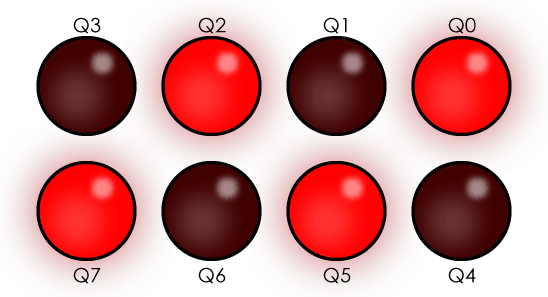b)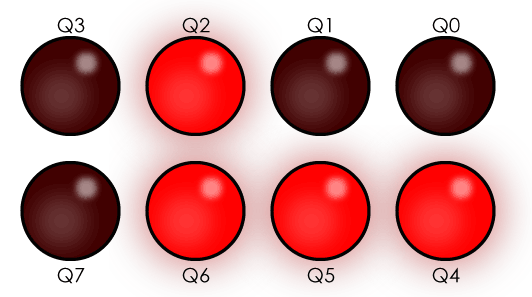2. Use the original `updateLEDs` to control specific LEDs attached to the shift register.
• Design 8-bit patterns and call `updateLEDs()` from the `loop()` function to reproduce these patterns of LEDs:
c)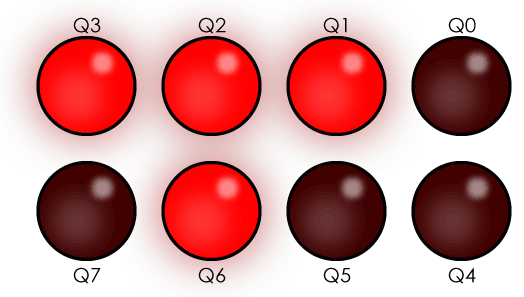d)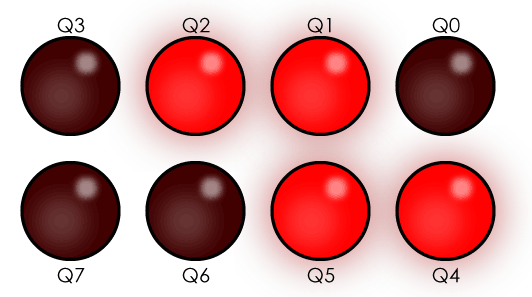Display all patterns the above patterns in one sketch. Call delay after each pattern so it can be seen clearly.

### Part 2: Chaining (2 marks)

With a partner, chain two shift registers together. Now your output will be 16 bits or 2 bytes long. Remember that one bit is shifted out on Q7' (pin 9), and you want it to shift that value into DS (pin 14) of the second shift register. In other words, connect pin 9 of the first register to pin 14 of the second shift register.

The following diagram shows this chaining: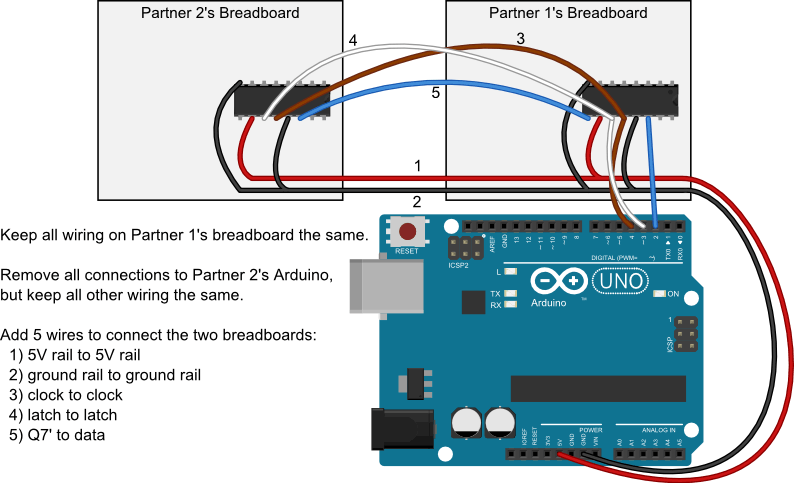There is a bit of a challenge in connecting both registers to the Arduino pins that control the clock and latch. Remember that the signals from the Arduino can be found along the bars to which you hook your clock and latch wires on Partner 1's breadboard.

Other specifications:

• Use `updateLEDsPattern()` pattern to set all 16 outputs of both shift registers from a 16 element array.
• Your lab instructor may request changes to specific LEDs, so once you have a stable pattern try controlling specific LEDs on each boart to understand where the LEDs are located,.
• use `updateLEDsPattern()` function in main to show three different patterns with a short delay between them.

### Part 3: 7 Segment Display Design (2 marks)

This exercise is to be completed alone after you are done with your Partner, but you can start designing while you wait if they aren't ready.

You will be given a Danger Shield. It has a shift register on it that controls a 7 segment display. Shift a sequence of 8-bit numbers into the Danger Shield's shift register one at a time using the original `updateLEDs()` function. The result should be at least three different and recognisable letters being shown on the 7 segment display one after the other - not including the F shown below.

Integration idea: you can rewrite `updateLEDs()` so that it takes and shifts an array of letters.

The shift register on the Danger Shield is connected to different pins than the First Build exercise. They are:

• data: 4
• clock: 8
• latch: 7

The lights on the 7 segment display correspond to these bits: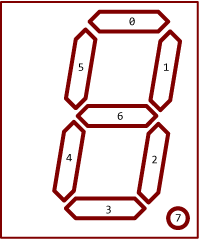Also note that the 7 segment display is grounding through the shift register - all the values are inverted, so you need to flip 1's to 0's and 0's to 1's in the pattern you would expect to write out.

For example if I wanted to display an F I would turn on these lights: 0, 4, 5, 6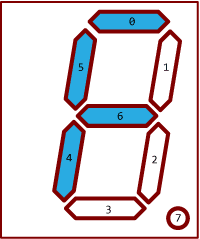This should be this binary value:

```        Binary value: 01110001
Digit pos'n:  76543210```

But it will be inverted, so we need to use binary 10001110, or decimal 128 + 8 + 4 + 2 = 142.

### Challenge

Note: This challenge uses the Danger Shield, so it should probably be done in-lab. If you think you can solve it without the shield, you can still show your work the next week.

Use the 7-segment display of your Danger Shield to indicate the rough position of one of the sliders.

• Get a reading from the slider.
• Use the map function to convert the reading to [0,9].
• Use the mapped reading as an array lookup to display the patterns in this array on the 7-segment display:
``````
int numberGlyphs[] =
{
B11000000, //0
B11111001, //1
B10100100, //2
B10110000, //3
B10011001, //4
B10010010, //5
B10000010, //6
B11111000, //7
B10000000, //8
B10011000, //9
B11111111, //10 - CLEAR
};
```
```